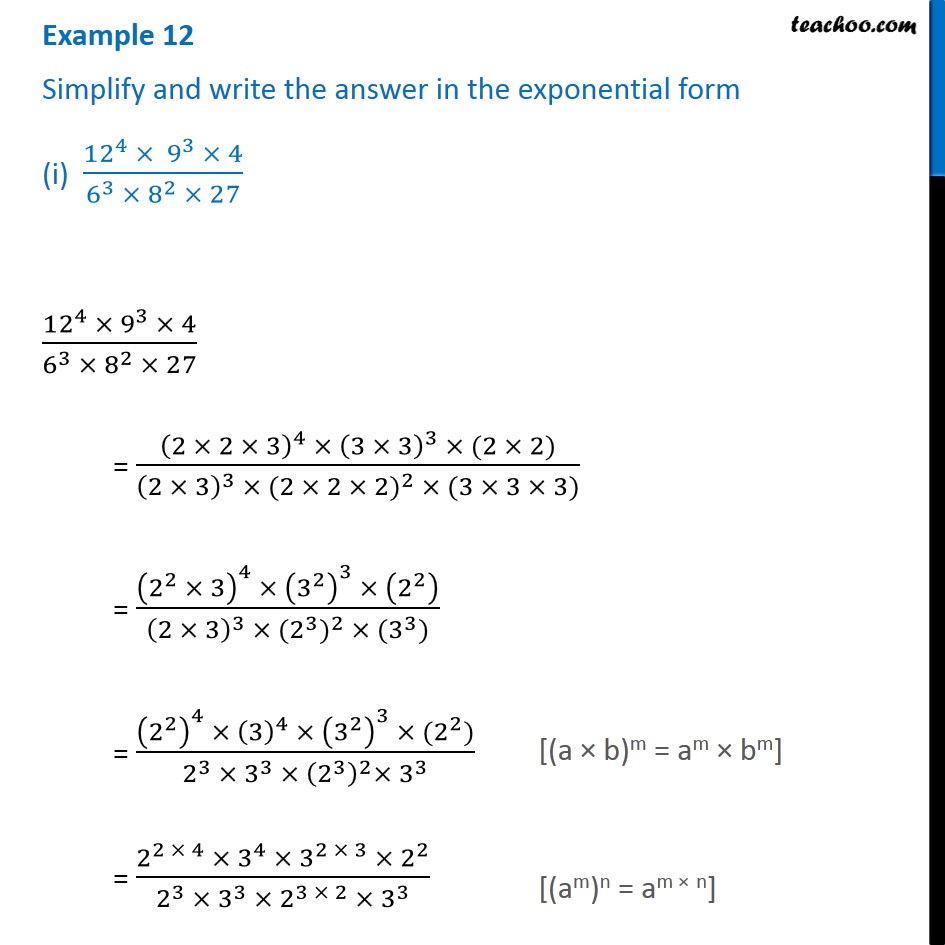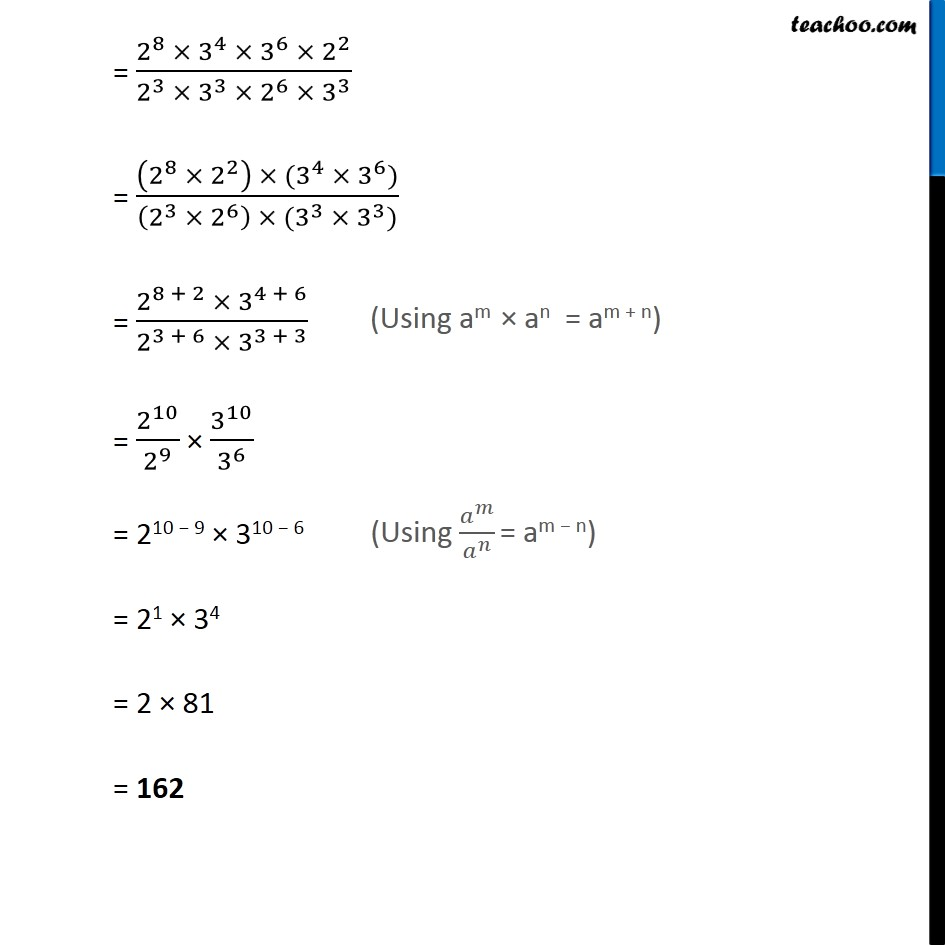1. Chapter 13 Class 7 Exponents and Powers
2. Concept wise
3. Law of exponents

Transcript

Example 12 Simplify and write the answer in the exponential form (i) (〖12〗^4 × 〖 9〗^3 × 4)/(6^3 ×〖 8〗^2 × 27) (〖12〗^4 × 9^3 × 4)/(6^3 × 8^2 × 27) = ((2 × 2 × 3)^4 × (3 × 3)^3 × (2 × 2))/((2 × 3)^3 × 〖(2 × 2 × 2)〗^2 × (3 × 3 × 3)) = ((2^2 × 3)^4 × (3^2 )^3 × (2^2 ))/((2 × 3)^3 × 〖(2^3)〗^2 × (3^3)) = ((2^2 )^4 × (3)^4 × (3^2 )^3 × (2^2))/(2^3 × 3^3 × (2^3 )^2×〖 3〗^3 ) = (2^(2 × 4) × 3^4 × 3^(2 × 3) ×〖 2〗^2)/(2^3 × 3^3 × 2^(3 × 2) × 3^3 ) [(a × b)m = am × bm] [(am)n = am × n] = (2^8 × 3^4 × 3^6 ×〖 2〗^2)/(2^3 × 3^3 × 2^6 × 3^3 ) = ((2^8 ×〖 2〗^2 ) × (3^4 × 3^6))/((2^3 × 2^6 ) × (3^3 × 3^3)) = (2^(8 + 2) × 3^(4 + 6))/(2^(3 + 6) ×〖 3〗^(3 + 3) ) = 2^10/2^9 × 3^10/3^6 = 210 − 9 × 310 − 6 = 21 × 34 = 2 × 81 = 162 (Using am × an = am + n) (Using 𝑎^𝑚/𝑎^𝑛 = am − n)